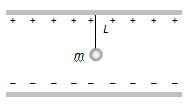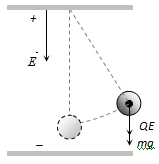# A small sphere carrying a charge ‘q’ is hanging in between two parallel plates by a string of length L. Time period of pendulum is . When parallel plates are charged, the time period changes to . The ratio  is equal toOption 1) Option 2) Option 3) Option 4) None of these

V Vakul

As we have learnt

when electric field applied downward direction -

- whereinNet downward force
Effect acceleration
Hence time period

Option 1)

Option 2)

Option 3)

Option 4)

None of these

Exams
Articles
Questions